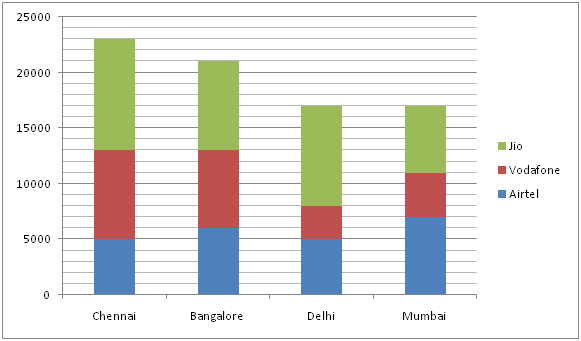# LIC HFL Associate/ Assistant Numerical Ability Questions – (Day-08)

Dear Aspirants, Our IBPS Guide team is providing new series of Numerical Ability Questions for LIC HFL Associate/ Assistant 2019 so the aspirants can practice it on a daily basis. These questions are framed by our skilled experts after understanding your needs thoroughly. Aspirants can practice these new series questions daily to familiarize with the exact exam pattern and make your preparation effective.

Check here for LIC HFL Mock Test 2019

Check here for IBPS RRB PO Mains Mock Test 2019

Check here for IBPS RRB Clerk Mains Mock Test 2019

### Click Here to Subscribe Crack High Level Puzzles & Seating Arrangement Questions PDF 2019 Plan

[WpProQuiz 7182]

Directions (01 – 05): What value should come in the place of (?) in the following number series?

1) 4080, 3360, 2730, 2184, 1716, ?

a) 1320

b) 1850

c) 1560

d) 1210

e) 1080

2) 3, 21, 33, 39, 39, ?

a) 52

b) 48

c) 33

d) 28

e) 22

3) 19, 17, 30, 82, 312, ?

a) 1528

b) 1236

c) 1328

c) 1124

e) 1456

4) 94, 95, 98, 104, 114, ?

a) 110

b) 116

c) 119

d) 124

e) 129

5) 2430, 1620, 1080, 720, ?, 320

a) 640

b) 560

c) 480

d) 680

e) 520

Directions (6 – 10): Study the following information carefully and answer the questions given below.

The given bar graph shows the number of person who uses the different networks in different cities.6) What is the average of the difference between the number of Airtel users in Chennai and Mumbai and the difference between the number of Vodafone users in Delhi and Bangalore?

a) 2000

b) 3000

c) 4000

d) 5000

e) None of these

7) The number of Jio users in Bangalore is approximately what percent of the total number of users in Mumbai all the networks together?

a) 43%

b) 45%

c) 47%

d) 49%

e) 41%

8) What is the average number of Vodafone users in all the cities together?

a) 5200

b) 5300

c) 5400

d) 5500

e) None of these

9) What is the ratio of the average number of Airtel and Jio users in Chennai to the average number of Vodafone and Jio users in Delhi?

a) 3: 2

b) 4: 3

c) 5: 4

d) 6: 5

e) None of these

10) In Bangalore, 60% of the Jio users are male and 80% of Airtel users are female. What is the difference between the male users in Jio and Airtel together and female users in Jio and Airtel together?

a) 1500

b) 2000

c) 3500

d) 3000

e) None of these

Directions (1-5) :

163 – 16 = 4080

153 – 15 = 3360

143 – 14 = 2730

133 – 13 = 2184

123 – 12 = 1716

113 – 11 = 1320

3 + (3 * 6) = 21

21 + (2 * 6) = 33

33 + (1 * 6) = 39

39 + (0 * 6) = 39

39 + (-1 * 6) = 33

19 * 1 – 2 = 17

17 * 2 – 4 = 30

30 * 3 – 8 = 82

82 * 4 – 16 = 312

312 * 5 – 32 = 1528

94 + 1 = 95

95 + 1 + 2 = 98

98 + 1 + 2 + 3 = 104

104 + 1 + 2 + 3 + 4 = 114

114 + 1 + 2 + 3 + 4 + 5 = 129

2430 ÷ 1.5 = 1620

1620 ÷ 1.5 = 1080

1080 ÷ 1.5 = 720

720 ÷ 1.5 = 480

480 ÷ 1.5 = 320

Directions (6-10) :

Difference of Airtel user in Chennai and Mumbai = 7000 – 5000 = 2000

Difference of Vodafone user in Delhi and Bangalore = 7000 – 3000 = 4000

Average = (2000 + 4000)/2 = 3000

Required percentage = 8000/(7000 + 4000 + 6000) * 100 = 47%

Average = (8000 + 7000 + 3000 + 4000)/4 = 5500

Average Airtel and Jio users in Chennai = (5000 + 10000)/2 = 7500

Average of Vodafone and Jio users in Delhi = (3000 + 9000)/2 = 6000

Required ratio = 7500: 6000 = 5: 4

Male user in Jio = 8000 * 60/100 = 4800

Female users in Jio = 8000 * 40/100 = 3200

Female users in Airtel = 80/100 * 6000 = 4800

Number of male users in Airtel = 20/100 * 6000 = 1200

Difference = (4800 + 3200) – (4800 + 1200) = 2000